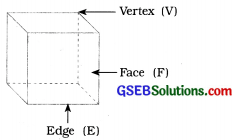# GSEB Class 8 Maths Notes Chapter 10 Visualising Solid

This GSEB Class 8 Maths Notes Chapter 10 Visualising Solid covers all the important topics and concepts as mentioned in the chapter.

## Visualising Solid Class 8 GSEB Notes

→ The figures having length and breadth only are known as 2-D figures.

→ It is very convenient to visualise these figures, e.g. Triangle, Circle, Square, Rectangle, etc.

→ Solids having length, breadth and height/ depth are known as 3-D figures.

→ It is slightly difficult to visualise shape of these figures, e.g. Cone, Cube, Sphere, Cylinder, etc.

→ A map depicts the location of a particular object / place in relation to other objects/places.

→ Different symbols are used to depict the different objects / places.

→ There is no reference or perspective in a map.

→ Map involves a scale which is fixed for a particular map.

→ Map reduce the real distances proportionately to distances on the paper.

→ Scale is a ratio between the drawing’s dimension to the actual dimension.→ A polyhedron is a solid shape which is bounded by polygons which are called its faces, these faces meet at edges which are line segments and the edges meet at vertices which are points.
Euler’s formula → F + V – E = 2→ Regular polyhedrons:
A polyhedron is regular, if its faces are made up of regular polygons and the same number of faces meet at each vertex, e.g. cube.

→ Prism:
A solid whose base and top are identical polygons and the sides are rectangle, is known as a prism.

→ Pyramid:
A pyramid is a polyhedron whose base is a polygon and whose other faces are triangles with a common vertex.